# About the derivation of Lorentz gauge condition

The question:
Show that the Lorentz condition ∂µAµ =0 is expressed as d∗ A =0.
Where A is the four-potential and * is the Hodge star, d is the exterior differentiation.

In four-dimensional space, we know that the Hodge star of one-forms are the followings.3. My attempt
Since the four potential one-form is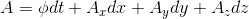Therefore we have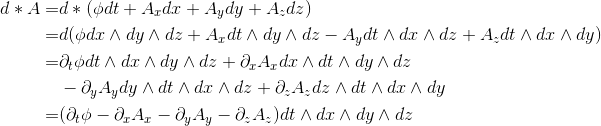Then d*A = 0 is equivalent of saying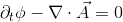(Where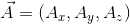)

However, the actual Lorentz gauge potential is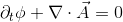(Where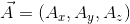)

I don't know why there is a sign difference?

#### Attachments

George Jones
Staff Emeritus
Gold Member
•QuantumRose
Aren't the indices "upstairs", here?
Opps, such a silly mistake........ thank you!

dextercioby